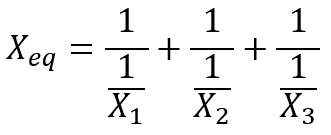Search
• Stu WØSTU

# Series & Parallel Component Calculations (G5C04)

The 2019-2023 General License exam pool requires some calculations of equivalent component values, such as a single component that is equivalent to multiple resistors in a parallel circuit arrangement. The details of this type of calculation are beneficial for the advanced Technician License student as well, who should understand how series and parallel circuit arrangements impact both voltage and current flow in circuits:

G5C04: What is the total resistance of three 100-ohm resistors in parallel?

A. .30 ohms B. .33 ohms C. 33.3 ohms D. 300 ohms

The General License Course section 6.3 is all about parallel and serial electronic components and the concept of equivalent component values. Based on Kirchoff’s Laws for electrical current and voltage, multiple electronic components such as resistors, capacitors, and inductors arranged in either parallel or serial connections in a circuit may be “replaced” by a singular equivalent component. Multiple General License exam pool questions test your mathematical skill in calculating the value of the equivalent component, or put another way, the equivalent value provided by the multiple components.

Each of the three component types mentioned above may be arranged in either series or parallel circuit configurations, and one of two general equation forms applies for calculating the equivalent component value depending on the type of component and the type of circuit arrangement. The calculations themselves are rather trivial once properly set up, so the real challenge of these questions boils down to applying the appropriate equation form among the six possible combinations of components and configuration.

Let’s consider the two general forms of the two equations involved, using a generic “X” component indicator. The X component in these equations may be replaced by R for resistance, C for capacitance, or L for inductance. (In no way is X intended to imply reactance, in these cases.)

Sum of Components form:...and so on for the number of components

Reciprocal of Reciprocals form:...and so on for the number of components

So, which of these two equations applies to each of the six combinations of component and connection types? Here’s how that shakes out:

 ​Component Type Connected in Series Connected in Parallel Resistor Sum of Components Reciprocal of Reciprocals Inductor Sum of Components Reciprocal of Reciprocals Capacitor Reciprocal of Reciprocals Sum of Components

Notice that resistors and inductors have identical equation form application, while capacitors are the opposite from those two. Keep this table of relationships in mind and you can’t go wrong in applying the proper equation to the scenario defined in the exam question.

Now, let’s apply the proper equation form to the question at hand, G5C04: What is the total resistance of three 100-ohm resistors in parallel? Here’s how that simple circuit arrangement may be depicted.Referencing the table above we see that parallel resistors’ equivalent resistance value is calculated using the reciprocal of reciprocal equation form, like this:From an intuitive standpoint, perhaps considering a ‘water flowing in pipes’ analogy to the circuit depiction above, this makes sense. In the parallel arrangement the total resistance to current flow offered by three equivalently sized pipes will be less than the resistance of any single pipe of the same size.Three resistors in a series connection arrangement would use the Sum of Components equation form.

If the three resistors were instead arranged in series, one behind the other and each offering additional resistance to the current flow in a single loop, the resistor values would add in accordance with the Sum of Components equation form above. [In the series arrangement scenario, total resistance would be 100+100+100 = 300 ohms.]

The answer to General Class question G5C04, What is the total resistance of three 100-ohm resistors in parallel? is “C. 33.3 ohms.”

198 views

See All